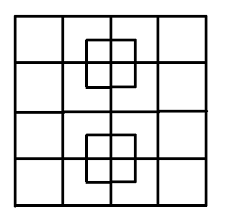Math | Recreation | How many squares can be found here

## How many squares can be found here?

### First approach: Lets simply count...

Well, here we have a $4\times 4$ square and two patches of $2\times 2$ squares. It is not difficult to see that a $4\times 4$ square contains sixteen different $1\times 1$ squares, nine $2\times 2$ squares, four $3\times 3$ squares and one $4\times 4$ square. When we sum up all these squares we get 16+9+4+1=30. Notice that $1^2+2^2+3^2+4^2=30$, this may give us a clue on the general pattern of the problem... Teacher need to emphasize that in mathematics we always look for patterns. Now, the two $2\times 2$ squares, located in the middle of the $4\times4$ square, have 4+1=5 squares each (or $1^2+2^2=5$). Hence the number of all different "hidden" squares is equal to 30+5+5=40.### Second approach: Lets find a general formula

By counting the squares we have seen that $1\times 1$ square contains 1 different square and that $2\times 2$ square contains $1^2+2^2=5$ different squares and that $3\times 3$ square contains $1^2+2^2+3^2=14$ different squares. Similarly, we have seen by counting that a $4\times 4$ square contains $1^2+2^2+3^2+4^2=30$ different squares. But what about $100\times 100$ square? How can we find the number of all different squares in $100\times 100$ square?
To answer this question, lets consider a $5\times 5$ square. If we take a $4\times 4$ square append the right most column with a column of four $1\times 1$ squares and append the last raw of the newly formed rectangle with a raw of five $1\times 1$ squares we get a $5\times 5$ square. Now, we already know that we can find $\sum_{i=0}^4 i^2=30$ different squares in a $4\times 4$ square. Lets find out how the additional appended column (without the appended raw) contributes to the number of different squares. Clearly, the appended column adds four $1\times 1$ squares. The appended column also adds three $2\times 2$ squares, we can see this by starting from the right top corner we start with a $2\times 2$ squares and count all the squares by moving one grid down. Similarly we can count all the additional $3\times 3$ squares formed by the appended column, this give us two different $3\times 3$ squares. Finally, in a similar way, we can count one $4\times 4$ square. Hence, the contribution of the appended column to the total sum of squares is equal to $\sum_{i=1}^4 i$. Similarly, the contribution of the appended raw (raw of five $1\times 1$ squares appended to the last raw of the rectangle) to the total sum of squares is equal to $\sum_{i=1}^5 i$. Summing up these two arithmetic sequences and adding them up yields $\frac{4\cdot (4+1)}{2}+\frac{5\cdot(5+1)}{2}=\frac{5\cdot (4+6)}{2} = 5^2.$ Hence, the total number of different squares that can be found in a $5\times 5$ square is equal to $\sum_{i=1}^5 i^2 = \frac{5\cdot (5+1)\cdot(2\cdot5+1)}{6} = 55.$ Notice that the above argument can be applied to $n\times n$ square. Hence, we can use induction to prove that the general formula for computing the sum of all different squares. For any integer $m$, the sum of all different squares that can be found in $m\times m$ square is equal to $\sum_{i=1}^m i^2= \frac{m(m+1)(2m+1)}{6}.$ Armed with this formula we can compute the number of all different squares "hidden" in $100\times 100$ square. The sum of all different squares in $100\times 100$ square is equal to $\frac{100\cdot 101\cdot 201}{6}=338350$.

Suppose we have a rectangle that consists out of $n\times m$ grid squares, where $n\le m$. How many different square can we find in this triangle? The question is very similar to the case of of the square. We may cut this triangle to a square consisting $n\times n$ grid square and a rectangle that consists out of $n \times (m-n)$ square. If we repeatedly append ($m-n$ times) a column of $n$ grid square to the right most column of the $n\times n$ square, then we get our $n\times m$ rectangle. Now, as we saw before, each time we append a column we increase the number of different square by $\sum_{i=1}^n$. Hence the number of all different squares that can be found on the $n\times m$ rectangle is equal to $\sum_{i=1}^n i^2+(m-n)\sum_{i=1}^n i = \frac{n(n+1)(2n+1)}{6}+ \frac{(m-n)(n^2+n)}{2}.$